## Practice Questions – Data Interpretation -2

Direction (1-4): Study the following graph carefully and answer the questions given below

Total number of Arts, Science and Commerce Students in Various colleges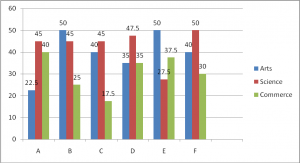1. The number of students taking Arts in college B, is how thousand fewer than the number of students taking Arts in college A and college C together?

(a) 12.5

(b) 27.5

(c) 50

(d) 10

(e) 62.5

(a) 12.5

Explanation:

Number of students taking Arts in College B= 50 thousand

Number of students taking Arts in college A and C = 22.5+40= 62.5 thousand

Required difference = (62.5-50) thousand= 12.5 thousand

2. In college F, the students taking commerce is what percent of the total number of students taking Arts, science and commerce in all?

(a) 45

(b) 55

(c) 59

(d) 70

(e) 25

(e) 25

Explanation:

In college F, number of students taking Arts, Science and commerce

= (40+50+30) thousand= 120 thousand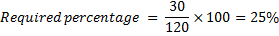3. How many candidates (in thousands) have taken commerce from all the colleges?

(a) 165

(b) 177

(c) 185

(d) 193

(e) 199

(c) 185

Explanation:

Number of students taking commerce in all colleges

= (40+25+17.5+35+37.5+30) thousand

= 185 thousand

4. What is the respective ratio of the number of the students taking science to the number of students taking Arts in college D?

(a) 14:19

(b) 12: 17

(c) 17: 19

(d) 19:14

(e) 13:18

(d) 19:14

Explanation:

Required ratio= 47.5: 35

= 19: 14

Directions (5-8): Study the following graph carefully and answer the questions given below it

Account of income and expenditure (in crores) of seven companies in the years 2000

Percentage Profit/loss=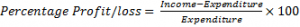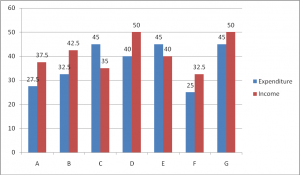5. Approximately, what is the percentage of profit/loss that companies C and D had together?

(a) 10% profit

(b) 12% profit

(c) 10% loss

(d) 12% loss

(e) There was no loss or profit

(e) There was no loss or profit

Explanation:

Total expenditure of Company C and D = 45+40= Rs.85 crores

Total income of company C and D = 35+50= Rs.85 crores

There is no profit no loss

6. If the income of company A had increased by 10% in year 2000 from year 1999 and profit earned in 1999 was 20% what was its expenditure in 1999?

(the value upto two decimal places in crores)

(a) 36.36

(b) 32.32

(c) 30.30

(d) Can’t be determined

(e) None of these

(e) None of these

Explanation:

Income of company A in 1999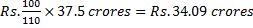Let expenditure in  1999 be Rs x crores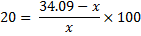0.2x = 34.09 –x

X= 28.40 crores

7. If the expenditure of Company G in 2000 was 20% more than its expenditure in the year 1999 and the Company has earned a profit of 10% in 1999. What was the company income in 1999 in crores Rs.?

(a) 37.5

(b) 41.25

(c) 34.09

(d) Can’t be determined

(e) None of these

(b) 41.25

Explanation:

Expenditure of Company G in 1999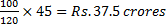Let its income in 1999 be 1 crores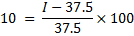3.75= I- 37.5

I= 37.5+3.75= Rs.41.25 crores

8. Which of the following Companies had the highest percentage of profit/loss in 2000?

(a) B

(b) C

(c) F

(d) A

(e) None of these

(d) A

Explanation:

Let us compare the ratio,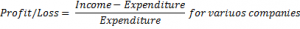Profit/Loss=  for variuos companies

A= 10/275

B= 10/32.5

C= -10/45

D= 10/40

E= -5/45

F= -7.5/25

G= 5/45

Now if we compare A, B, C and D obviously 10/27.5 is the grestest, because numerator is the same while denominator is the least in case of A